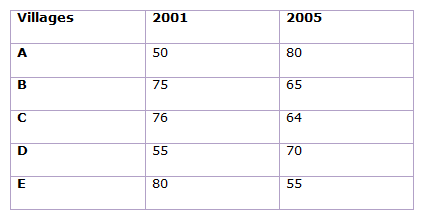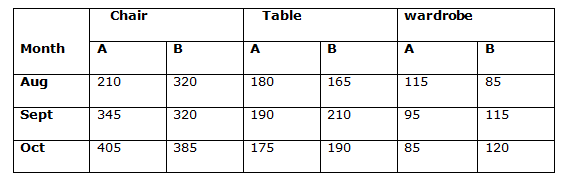# Quantitative Aptitude Questions (Data Interpretation) for RRB PO Mains 2018 Day-198

Dear Readers, RRB is conducting Online Examination for the recruitment of PO. To enrich your preparation here we have providing new series of Data Interpretation – Quantitative Aptitude Questions. Candidates those who are appearing in RRB PO Mains Exams can practice these Quantitative Aptitude average questions daily and make your preparation effective.

[WpProQuiz 3700]

Click “Start Quiz” to attend these Questions and view Solutions

Directions (1-5): Study the following information and answer the questions that follow:

There are two companies namely A and B, which sell chairs, tables and wardrobes in 3 months August, September and October. The ratio of chair, tables and wardrobes sold by A in August is 42: 36: 23 while ratio of chairs sold by A in August, September and October is 14: 23: 27. Wardrobes sold by A in August is 230 less than chairs sold in September by A. In September 665 chairs, 400 tables and 210 wardrobes were sold by two companies together. B sold same number of chairs in Aug and September. Number of tables sold by company B in September was equal to number of chairs sold by A in August while number of wardrobes sold by A in August and B in September were equal. Company B sold total 1025 chairs in these three months together which was 480 more than total number of tables sold by A. Ratio of tables sold by A and B in August is 12: 11 and in October is 35: 38 respectively. Total number of items sold in August was 1075. Total number of wardrobes sold by A in October was 35 less than wardrobe sold by B in October, while sum of wardrobe sold by A and B in October is 205.

1) What is the ratio of number of tables sold by A in August to that of B in September?

a) 7:6

b) 6:7

c) 12:13

d) 11:12

e) None of these

2) Find the number of wardrobes sold by B in October.

a) 80

b) 120

c) 115

d) 95

e) 125

3) Find the difference in number of chairs sold by A and B in August.

a) 100

b) 120

c) 105

d) 110

e) 112

4) Total number of chairs sold by B in September and October is

a) 750

b) 725

c) 705

d) 715

e) 405

5) By what percent tables sold by A in October are more than wardrobes sold by B in October?

a) 83%

b) 54%

c) 67%

d) 56%

e) None of these

Directions (6-10): The Table given below shows the percentage of valid voters in 5 villages in two years 2001 and 2005. Study the graph carefully to answer the based questions.

NOTE- Total voters in any year = Valid voters + Invalid voters6) What is the difference between invalid voters of village C in the two given years if valid voters in 2005 in that village are 4000 and the ratio of valid voters of village C in 2001 to 2005 is 19: 25?

a) 1190

b) 1250

c) 1290

d) 1350

e) 1365

7) If in village A in 2005, 2500 voters were declared invalid voters 10% of valid voters opted NOTA and the winner got 200 more votes than losing candidate, then find the total vote that losing candidate got in 2005 in village A.

a) 4400

b) 4600

c) 5400

d) 5200

e) 4800

8) In village B if the ratio of total voters in 2001 to 2005 is 26: 23, then find the ratio of invalid voters in 2001 to the invalid voters in 2005 in same village.

a) 131: 160

b) 130: 161

c) 127: 141

d) 18: 35

e) None of these

9) If there are 1600 males in valid voters of village E in 2001 and the females in valid voters of same village and same year contributed is 36% of total valid voters, then find the percentage of invalid male voters in total population if total males in village E in 2001 were 2000.

a) 2%

b) 4%

c) 6%

d) 8%

e) 8%

10) If the ratio of valid voters of village B in 2001 and invalid voters of village D in 2005 was 16: 3, then total voters of village D in 2005 were what percent more or less than those of village B in 2001?

a) 45 2/7 %

b) 53 4/5 %

c) 53 1/8 %

d) 52 1/8 %

e) 50 2/5 %

Directions (1-5):

Let number of chairs, tables and wardrobes sold by A in August be 42x, 36x and 23x. Also, let chairs sold by A in August, September and October be 14y, 23y and 27y respectively.

∴42x = 14y ⇒y = 3x

and, 23x = 23y – 230

⇒x = 5 and y = 15

Now,

Chairs sold by B in September = 665 – 345 = 320

Chairs sold by B in August = 320

Tables sold by B in September = Chairs sold by A in Aug = 210

∴Table sold by A in September = 400 – 210 = 190

Wardrobes sold by B in September = wardrobes sold by A in Aug = 115

∴Wardrobes sold by A in September = 210 – 115 = 95

Chairs sold by B in October = 1025 – 320 – 320 = 385

Tables sold by A in October = (1025 – 480) – (180 + 190) = 175

Tables sold by B in August = (11/12) × 180 = 165

Tables sold by B in October = (38/35) × 175 = 190

Wardrobes sold by B in August = 1075 – (210 + 320 + 180 + 165 + 115) = 85

Let wardrobes sold by A in October be a and that by B be b in October

∴a = b – 35 and a + b = 205

⇒a = 85 and b = 120Required Ratio = 180/210 = 6/7

Wardrobes sold by B in Oct = 120

Required difference = 320 – 210 = 110

Total chairs sold by B in September and October = 320 + 385 = 705

[(175-120)/120] * 100 = 45.83%

Direction (6-10) :

Invalid voter of village C in 2005 = 4000 * 36/64 = 2250

Valid voter of village C in 2001 = 4000 * 19/25 = 3040

Now, invalid voter of village C in 2001 = 3040 * 24/76 = 960

∴Required diff. = 2250 – 960 = 1290

Total valid votes of village A in 2005 = 2500 * 100/20 * 80/100 = 10000

Total valid votes excluding Nota in village A in 2005 = 10000 * 90/100 = 9000

∴According to question,

x + (x + 200) = 9000

⇒x = 4400

Required no. of votes of losing candidates = 4400

Let the total voters in 2005 are x.

Total voters in 2001 = (26/23) x

∴ [x * 26/23 * 25/100] / [x * 35/100] = 130/161

Total invalid male of village E in 2001

= 2000 – 1600 = 400

Total valid voters in 2001 = 1600 * 100/64 = 2500

Total Voters in 2001 = 2500 * 100/80 = 3125

∴ required % = (400/3125) * 100 = 12.8 %

Let total voters of village B in 2001 = x

& Total voters of village D in 2005 = y

∴ [x * 75/100]/ [y * 30/100] = 16/3

x/y = 32/15

Required % = 17/32 * 100 = 53 1/8 %

Daily Practice Test Schedule | Good Luck

 Topic Daily Publishing Time Daily News Papers & Editorials 8.00 AM Current Affairs Quiz 9.00 AM Quantitative Aptitude “20-20” 11.00 AM Vocabulary (Based on The Hindu) 12.00 PM General Awareness “20-20” 1.00 PM English Language “20-20” 2.00 PM Reasoning Puzzles & Seating 4.00 PM Daily Current Affairs Updates 5.00 PM Data Interpretation / Application Sums (Topic Wise) 6.00 PM Reasoning Ability “20-20” 7.00 PM English Language (New Pattern Questions) 8.00 PM